# Test Prep Plan - Take a practice test

Take this practice test to check your existing knowledge of the course material. We'll review your answers and create a Test Prep Plan for you based on your results.
How Test Prep Plans work
1
2Based on your results, we'll create a customized Test Prep Plan just for you!
3Study smarter
Study more effectively: skip concepts you already know and focus on what you still need to learn.

# Properties of Triangles: Tutoring Solution Chapter Exam

Exam Instructions:

Choose your answers to the questions and click 'Next' to see the next set of questions. You can skip questions if you would like and come back to them later with the yellow "Go To First Skipped Question" button. When you have completed the practice exam, a green submit button will appear. Click it to see your results. Good luck!

### Page 1

#### Question 2 2. What type of triangle is pictured?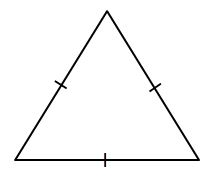#### Question 3 3. What type of triangle is pictured?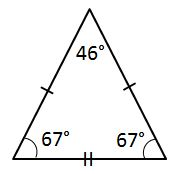#### Question 4 4. Given the triangle PQE, calculate the measure of Angle Q.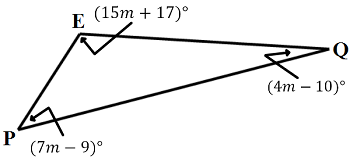### Page 2

#### Question 7 7. What pictured shape do we draw to identify the measured length of a triangle's side?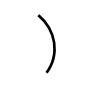#### Question 8 8. Given the triangle ABC, solve for the value of y.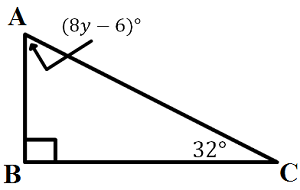#### Question 9 9. What is the area of ABCDE, as shown in the diagram?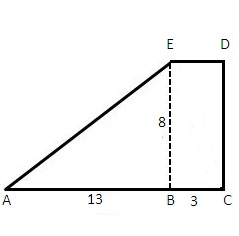#### Question 10 10. What type of triangle is pictured?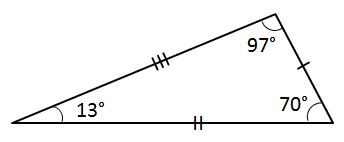### Page 3

#### Question 11 11. Josh claims that these triangles are similar by SSS. Do you agree or disagree with Josh?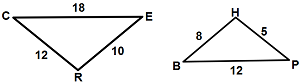#### Question 14 14. If angle a is 60 degrees, what is the measure of angle b?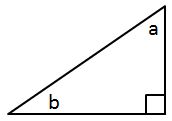### Page 4

#### Question 16 16. In the pictured triangle, identify the altitude(s).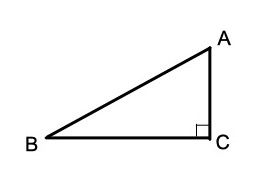#### Question 17 17. If angle a is 68 degrees and angle b is 37 degrees, what is the measure of angle c?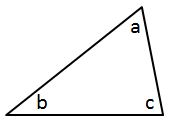#### Question 19 19. What type of triangle is pictured?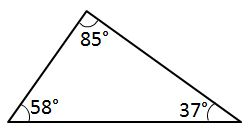#### Question 20 20. In the pictured triangle, what is the centroid?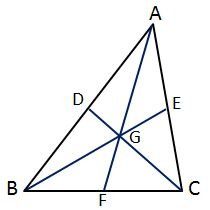### Page 5

#### Question 22 22. What is the area of the pictured rectangle?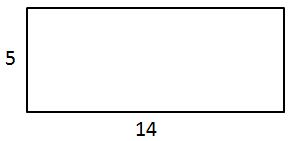#### Question 23 23. If angle a is 66 degrees and angle b is 54 degrees, what is the measure of angle d?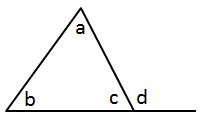#### Question 24 24. In the pictured triangle, which of the following lines is an altitude?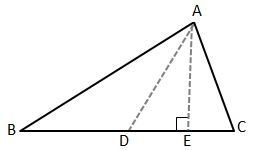#### Question 25 25. In the pictured triangle, which of these is a median?### Page 6

#### Question 27 27. Conclude whether triangle BEN is similar to triangle MAT.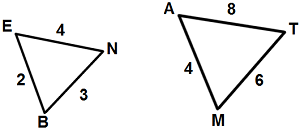#### Question 28 28. What is the area of the pictured triangle?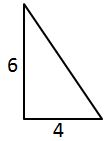#### Question 29 29. In triangle GBN, Angle G is seven more than three times a number, Angle B is five more than four times the number, and Angle N is twelve less than five times the number. Solve for the measure of Angle G.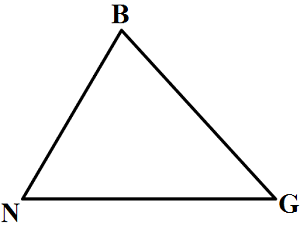#### Properties of Triangles: Tutoring Solution Chapter Exam Instructions

Choose your answers to the questions and click 'Next' to see the next set of questions. You can skip questions if you would like and come back to them later with the yellow "Go To First Skipped Question" button. When you have completed the practice exam, a green submit button will appear. Click it to see your results. Good luck!

Support